Courses

# The Force Method of Analysis: Frames - 1 Civil Engineering (CE) Notes | EduRev

## Civil Engineering (CE) : The Force Method of Analysis: Frames - 1 Civil Engineering (CE) Notes | EduRev

The document The Force Method of Analysis: Frames - 1 Civil Engineering (CE) Notes | EduRev is a part of the Civil Engineering (CE) Course Structural Analysis.
All you need of Civil Engineering (CE) at this link: Civil Engineering (CE)

Instructional Objectives

After reading this chapter the student will be able to
1. Analyse the statically indeterminate plane frame by force method.
2. Analyse the statically indeterminate plane frames undergoing support settlements.
3. Calculate the static deflections of a primary structure (released frame) under external loads.
4. Write compatibility equations of displacements for the plane deformations.
5. Compute reaction components of the indeterminate frame.
6. Draw shear force and bending moment diagrams for the frame.
7. Draw qualitative elastic curve of the frame.

Introduction

The force method of analysis can readily be employed to analyze the indeterminate frames. The basic steps in the analysis of indeterminate frame by force method are the same as that discussed in the analysis of indeterminate beams in the previous lessons. Under the action of external loads, the frames undergo axial and bending deformations. Since the axial rigidity of the members is much higher than the bending rigidity, the axial deformations are much smaller than the bending deformations and are normally not considered in the analysis. The compatibility equations for the frame are written with respect to bending deformations only. The following examples illustrate the force method of analysis as applied to indeterminate frames.

Example 11.1

Analyse the rigid frame shown in Fig.11.1a and draw the bending moment diagram. Young’s modulus E and moment of inertia I are constant for the plane frame. Neglect axial deformations.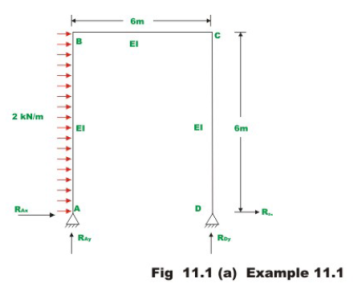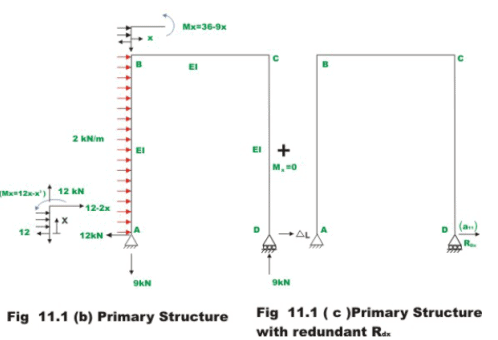The given one- storey frame is statically indeterminate to degree one. In the present case, the primary structure is one that is hinged at A and roller supported at D. Treat horizontal reaction at D, RDx as the redundant. The primary structure (which is stable and determinate) is shown in Fig.11.1.b.The compatibility condition of the problem is that the horizontal deformation of the primary structure (Fig.11.1.b) due to external loads plus the horizontal deformation of the support , due to redundant RDx (vide Fig.11.1.b) must vanish. Calculate deformation a11 due to D in the direction of RDx . Multiplying this deformation a11 with RDx , the deformation due to redundant reaction can be obtained.

Δ = a11 RDx                        (1)

Now compute the horizontal deflection ΔL and D due to externally applied load. This can be readily determined by unit load method. Apply a unit load along RDx as shown in Fig.10.1d.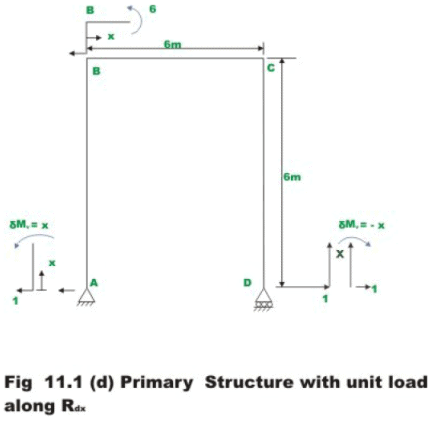The horizontal deflection ΔL at D in the primary structure due to external loading is given by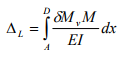(2)

where δMv is the internal virtual moment resultant in the frame due to virtual load applied at D along the resultant and RDx and M is the internal bending moment of the frame due to external loading (for details refer to Module 1,Lesson 5).Thus,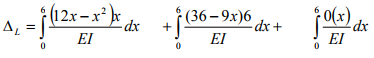(span AB, origin at A)        (span BC, origin at B)      (span DC, origin at D)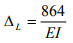(3)

In the next step, calculate the displacement a11 at D when a real unit load is applied at D in the direction of RDx (refer to Fig.11.1 d). Please note that the same Fig. 11.1d is used to represent unit virtual load applied at D and real unit load applied at D. Thus,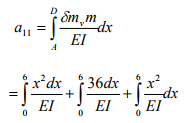= 360/EI                                (4)

Now, the compatibility condition of the problem may be written as

ΔL + a11RDx = 0                        (5)

Solving,

RDx = −2.40 kN                          (6)

The minus sign indicates that the redundant reaction RDx acts towards left. Remaining reactions are calculated from equations of static equilibrium.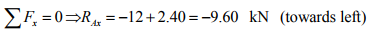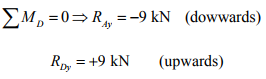The bending moment diagram for the frame is shown in Fig. 11.1e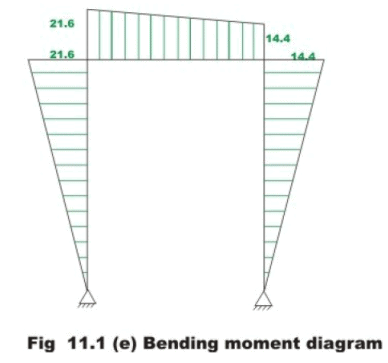Example 11.2
Analyze the rigid frame shown in Fig.11.2a and draw the bending moment and shear force diagram. The flexural rigidity for all members is the same. Neglect axial deformations.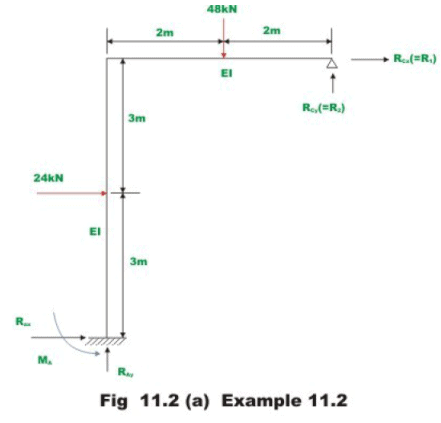Five reactions components need to be evaluated in this rigid frame; hence it is indeterminate to second degree. Select Rcx (=R1) and Rcy (=R2) as the redundant reactions. Hence, the primary structure is one in which support A is fixed and the support C is free as shown in Fig.11.2b. Also, equations for moments in various spans of the frame are also given in the figure.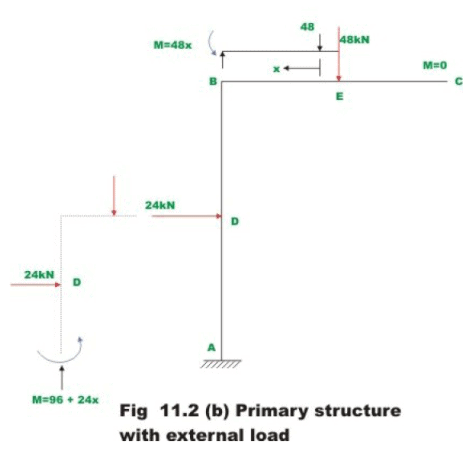Calculate horizontal (ΔL)and vertical (ΔL)2 deflections at C in the primary structure due to external loading. This can be done by unit load method. Thus,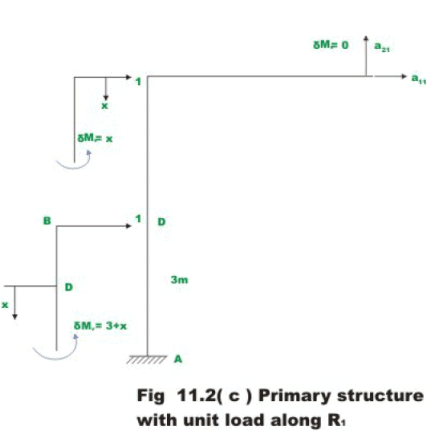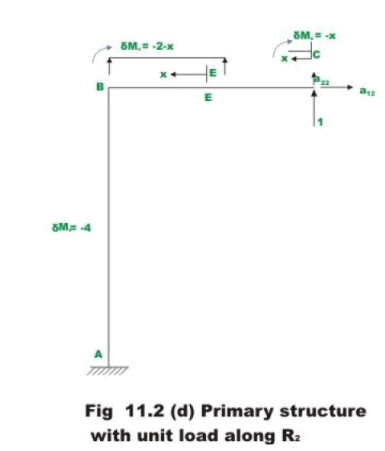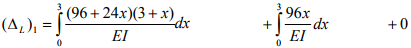(Span DA, origin at D)                 (Span BD, origin at B                (span BC, Origin B)

= 2268/EI                                     (1)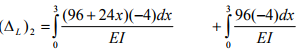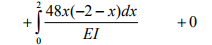(Span DA, origin at D)          (Span BD, origin at B)     (Span BE, origin at E)       (Span EC, Origin C)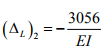(2)

In the next step, evaluate flexibility coefficients, this is done by applying a unit load along, R1 and determining deflections a11 and a21 corresponding to R1 and R2 respectively (vide, Fig .11.2 c). Again apply unit load along R2 and evaluate deflections a22 and a12 corresponding to R2 and R1 and respectively (ref. Fig.11.2d).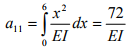(3)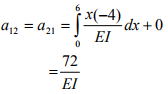(4)

and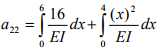= 117.33/EI                                    (5)

In the actual structure at C, the horizontal and vertical displacements are zero .Hence, the compatibility condition may be written as,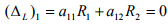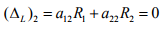(6)

Substituting the values of (ΔL)1, (Δl)2, a11, a12 in the above equations and solving for and R1, R2 we get

R1 = -1.056 kN (towards left)
R2 = 27.44 kN (upwards)

The remaining reactions are calculated from equations of statics and they are shown in Fig 11.2e. The bending moment and shear force diagrams are shown in Fig. 11.2f.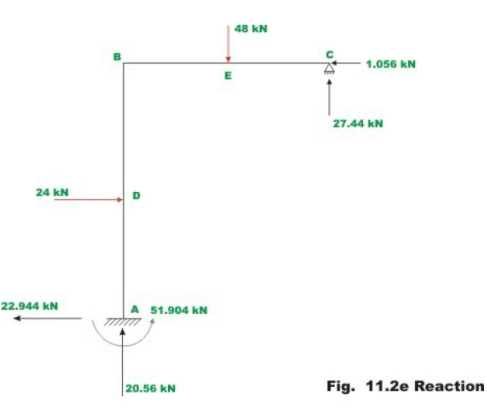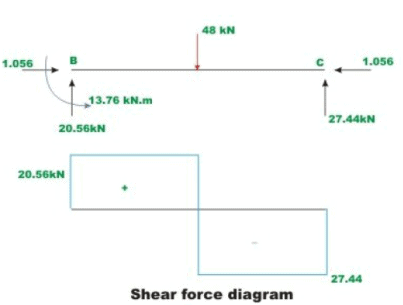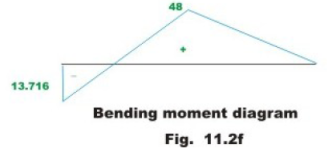Offer running on EduRev: Apply code STAYHOME200 to get INR 200 off on our premium plan EduRev Infinity!

## Structural Analysis

30 videos|122 docs|28 tests

,

,

,

,

,

,

,

,

,

,

,

,

,

,

,

,

,

,

,

,

,

;